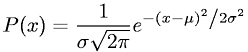Equations > Statistics > Distributions > Gaussian Normal Distribution

### Gaussian Normal DistributionLatex Code:

MathML Code:

 $P\left(x\right)=\frac1\sigma \sqrt{2\pi }{e}^{-{\left(x-\mu \right)}^{2}\mathord/\vphantom-{\left(x-\mu \right)}^{2}2{\sigma }^{2}2{\sigma }^{2}}$

MathType 5.0: